# What’s not in the power MOSFET data sheet, part 1: temperature dependency

Other Parts Discussed in Post: CSD17576Q5B, CSD19532Q5B

Power metal-oxide semiconductor field-effect transistor (MOSFET) data sheets provide useful information such as key specifications, ratings and characteristics to help you confirm that the device will operate as intended. You may have questions about how a parameter varies, however, so in this article I’ll explain not just what’s in the data sheet but more importantly, what’s not.

MOSFET data-sheet review

Let’s use the TI CSD17576Q5B NexFET™ data sheet as an example. The first page, shown in Figure 1, summarizes of the device capabilities and is divided into features, applications and description sections, including a schematic illustration of the FET package.

The first page also includes product summary, ordering information and absolute maximum ratings tables. The product summary table is a snapshot of typical parameters so that you can pick the right FET for your application. Ordering information is self-explanatory. The absolute maximum ratings table lists the boundaries for safe operation, outside of which the MOSFET could be permanently damaged. Unless otherwise noted, the specifications and ratings in these tables are all at an ambient temperature, TA = 25°C. In addition, typical performance plots of RDS(on) vs. VGS (at case temperatures of TC = 25°C and 125°C) and gate charge are also part of the first page.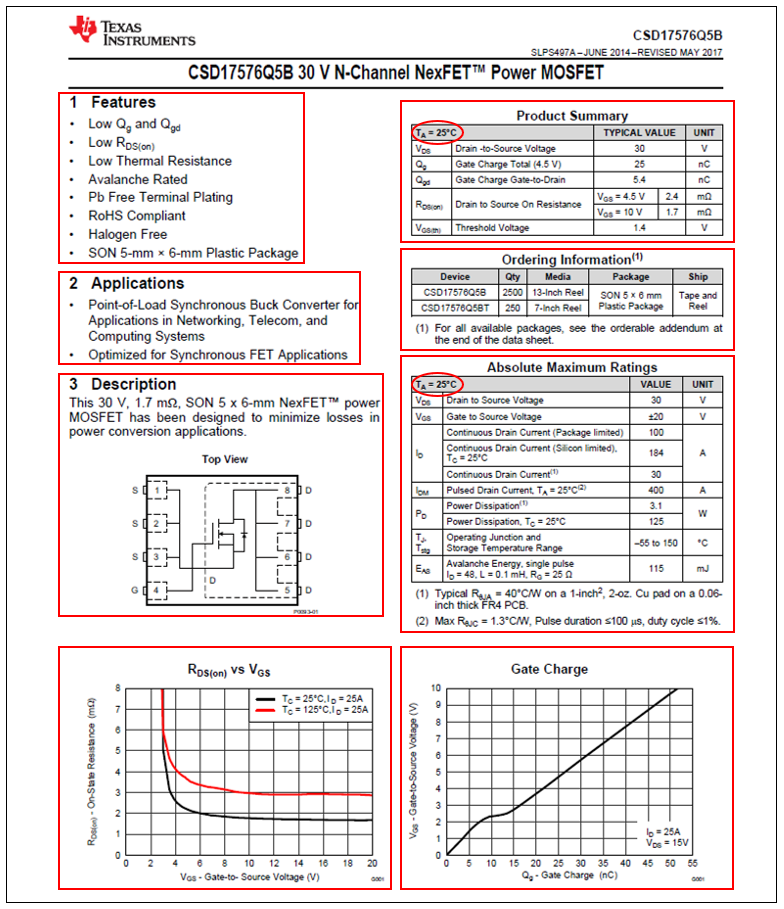Figure 1: First page of the CSD17576Q5B NexFET™ data sheet

The second page of the data sheet includes the table of contents and the revision history. Next are the specifications tables, electrical characteristics and thermal information, followed by graphs displaying the typical MOSFET characteristics. Then there is a section on device and documentation support. The data sheet includes the mechanical, packaging and orderable information in its final section. Unless otherwise noted, all specifications and ratings are at an ambient temperature, TA = 25°C.

Temperature dependence

Some of the FET specifications in the absolute maximum ratings table are temperature-dependent, including the drain-to-source voltage (VDS), continuous drain current (ID), pulsed drain current (IDM) and power dissipation (PD). The maximum VGS ratings guarantee that there is no gate-oxide breakdown during operation and is temperature-independent. Avalanche energy (EAS) is tested at case temperatures of TC = 25°C and TC = 125°C, with a corresponding graph in the typical MOSFET characteristics graph showing a reduction in EAS at elevated temperatures.

Static characteristics

The electrical characteristics table is broken down into static, dynamic and diode characteristics, as shown in Figure 2. Let’s look at the temperature-dependent FET parameters in the static characteristics section: the temperature variation of drain-to-source breakdown voltage (BVDSS), drain-to-source leakage current (IDSS), gate-to-source leakage current (IGSS) and transconductance (gfs) are not included in the data-sheet graphs. The typical MOSFET characteristics graph does include the threshold voltage, (VGS(th)) and on-resistance (RDS(on)) vs. temperature. The threshold voltage has a negative temperature coefficient and the on-resistance has a positive temperature coefficient.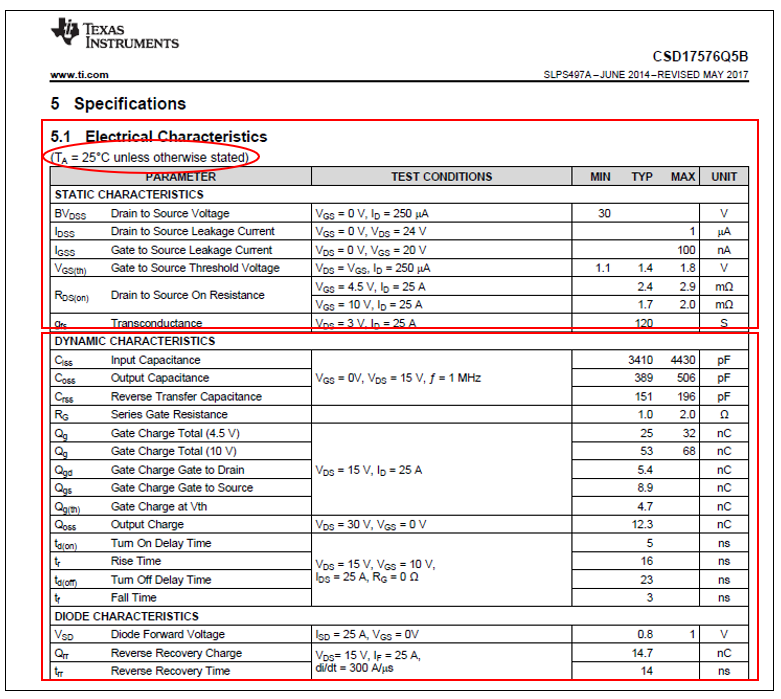Figure 2: The electrical characteristics table in the CSD17576Q5B NexFET™ data sheet

Figure 3 is the temperature variation of BVDSS for two power MOSFETs: the CSD17576Q5B 30-V trench FET and the CSD19532Q5B 100-V superjunction device; the curves in Figure 3 show the temperature dependence for BVDSS as well as IDSS and IGS. As the temperature increases, the breakdown voltage for both increases nearly linearly. The slope of the line is the positive temperature coefficient of BVDSS and will differ based on the FET’s process technology and voltage rating. Notice that the positive temperature coefficient is less for the CSD19532Q5B than for the CSD17576Q5B.Figure 3: Normalized BVDSS vs. temperature: CSD17576Q5B (a); CSD19532Q5B (b)

Figure 4 shows the temperature dependence of IDSS for the CSD17576Q5B and CSD19532Q5B. The lower-voltage FET, the CSD17576Q5B, displays more variation over the temperature range from -55°C to 150°C. For both devices, the plots tend to flatten out at low temperatures. This is not actual behavior but a test measurement system limitation at the very small currents being measures. The device physics dictate a continual downward trend at low temperatures.Figure 4: Normalized IDSS vs. temperature: CSD17576Q5B (a); CSD19532Q5B (b)

As shown in Figure 5 for the CD17576Q5B and CSD19532Q5B, IGSS also has a positive temperature variation. The relative increase in IGSS is greater for the CSD19532Q5B over the temperature range from -55°C to 150°C. Again, the flattening of the curves at low temperatures is caused by the resolution of the test measurement system.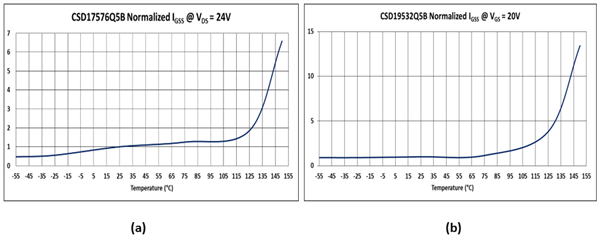Figure 5: Normalized IGSS vs. temperature: CSD17576Q5B (a); CSD19532Q5B (b)

The last parameter, gfs, is also temperature-dependent. You can use the transfer curves from the CSD17576Q5B and CSD19532Q5B data sheets as shown in Figure 6 to estimate gfs using Equation 1:

gfs = ΔIDS/ΔVGS                    (1)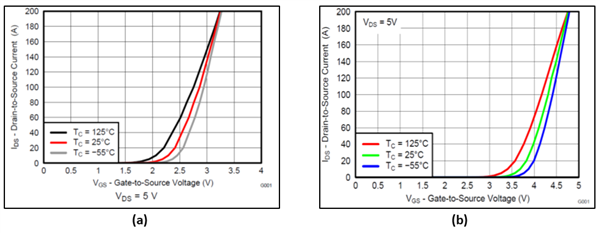Figure 6: Transfer characteristics: CSD17576Q5B (a); CSD19532Q5B (b)

Picking data points from the data-sheet curves, Table 1 lists the estimated values for gfs. You can see that transconductance has a negative temperature coefficient.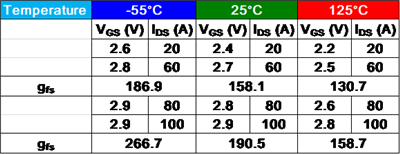Table 1: Estimated gfs values for the CSD17576Q5B

You can make the same gfs estimates using the transfer characteristics for the CSD19532Q5B, as listed in Table 2.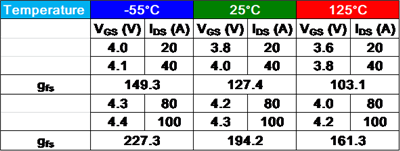Table 2: Estimated gfs values for the CSD19532Q5B

Dynamic characteristics

Parameters in the dynamic characteristics section are an indication of the MOSFET’s switching speed. These include the parasitic capacitances (CISS, COSS and CRSS), the internal series gate resistance (RG) and the charge parameters (QG, QGD, QGS and QOSS). These parameters, along with the external gate-drive circuit, determine the typical switching times (td(on), tr, td(off) and tf). There is minimal temperature variation of the parasitic capacitances and charge parameters. RG varies with temperature but is typically swamped out by an external gate resistor and the output impedance of the gate driver, resulting in some minor deviation of the switching times specified in the data sheet. Figure 7 shows a MOSFET with the parasitic capacitances and internal series gate resistance.Figure 7: MOSFET model with parasitic elements

Diode characteristics

The last section of the electrical characteristics table is the drain-to-source body-diode specifications. The diode forward voltage (VSD) has a negative temperature characteristic, as shown in typical MOSFET characteristics. Reverse-recovery charge (Qrr) and reverse-recovery time (trr) both increase at elevated temperatures. Because of this, reverse-recovery losses also increase at elevated temperatures.

Figure 8 shows the reverse-recovery behavior with temperature for two non-TI FETs. Qrr is the area enclosed by the drain current and trr is the time it takes for the current to return to zero. You can expect similar behavior from TI NexFET devices over temperature.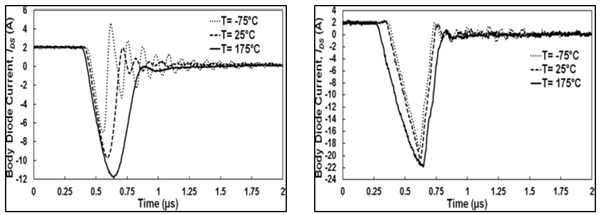Figure 8: Reverse-recovery current vs. temperature for two FETs

Safe operating area

Engineers often ask me how to derate for temperature from the safe operating area (SOA) curves in a MOSFET data sheet. Figure 9 shows the SOA curves at TA = 25°C for the CSD17576Q5B and CSD19532Q5B.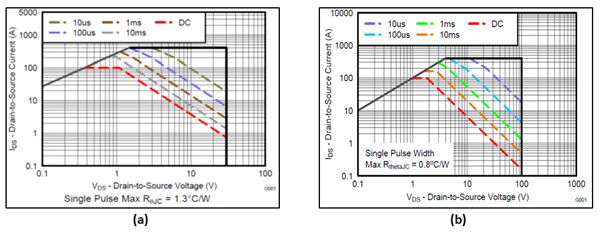Figure 9: Maximum safe operating area at TA = 25°C: CSD17576Q5B (a); and CSD19532Q5B (b)

The easiest approach is to use a linear derating factor. From the graph, determine the SOA current, IDS(SOA), at the voltage, VDS(SOA) and the pulse width of interest. Equation 2 calculates the SOA current at temperature T (°C) as:

IDS(SOA@T) = IDS(SOA) × (TJmax - T)/(TJmax - 25°C)                             (2)

Equation 2 yields 0 current when T = TJmax, specified in the data sheet.

Conclusion

In this technical article, I reviewed a TI NexFET data sheet, what’s in it and what’s not. I explored specifications that have a temperature dependency not included in the data sheet and provided typical curves and data showing how these specifications may vary with temperature. The examples used in this article were for two specific TI NexFET devices and showed the general trends versus temperature.

The typical curves presented in this article are to help you understand how these parameters vary with temperature, but they are no guarantee of actual performance. Always use the data-sheet limits when designing with TI FETs. If you don’t see certain specifications in the data sheet, please request them from TI in the E2E forum.

Additional resources

Reference

1. Jahdi, Saeed, Olayiwola Alatise, Roozbeh Bonyadi, Petros Alexakis, Craig Fisher, Jose A. Ortiz Gonzalez, Li Ran, and Philip Mawby “An Analysis of the Switching Performance and Robustness of Power MOSFETs Body Diodes: A Technology Evaluation.” IEEE Transactions on Power Electronics 30, no. 5 (May 2015): pp. 2383-2394.